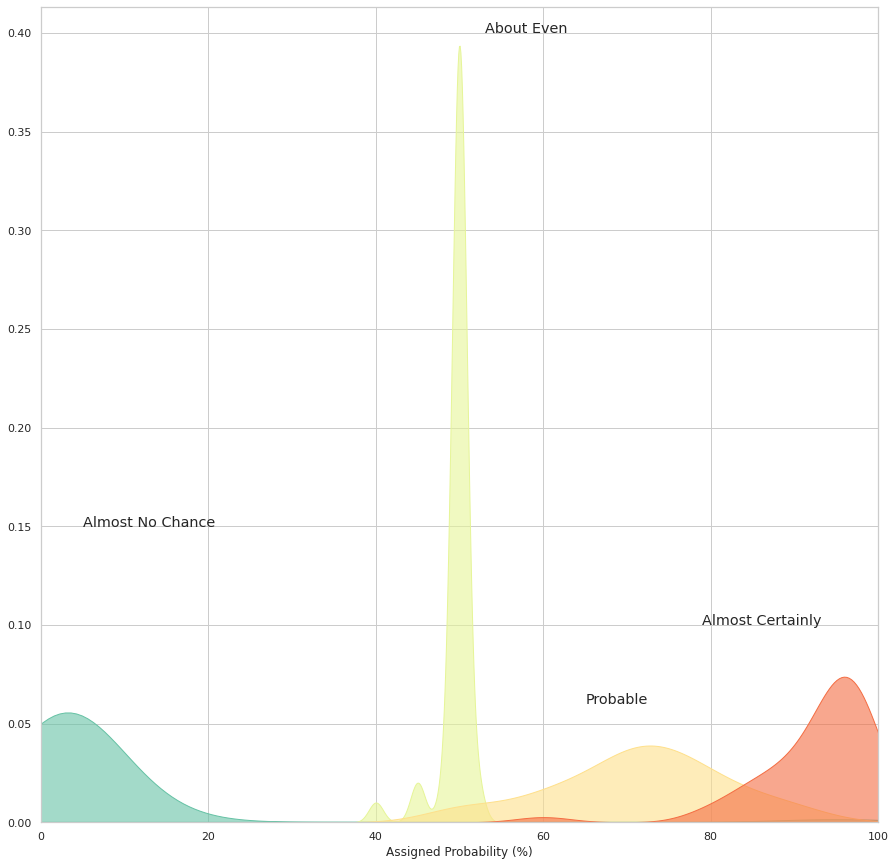# How probable is 'probable'?

Oct 04, 2021

## Probability

Probability is a measure that is associated with how certain we are of outcomes of a particular experiment or activity. An experiment is a planned operation carried out under controlled conditions. If the result is not predetermined, then the experiment is said to be a chance experiment. Flipping one fair coin twice is an example of an experiment.

A result of an experiment is called an outcome. The sample space of an experiment is the set of all possible outcomes. Three ways to represent a sample space are: to list the possible outcomes, to create a tree diagram, or to create a Venn diagram. The uppercase letter S is used to denote the sample space. For example, if you flip one fair coin, S = { H , T } where H = heads and T = tails are the outcomes.

An event is any combination of outcomes. Upper case letters like A and B represent events. For example, if the experiment is to flip one fair coin, event A might be getting at most one head. The probability of an event A is written P ( A ).

## How probable is ‘probable’?

The analysis started with a report about the probability of an invasion of Yugoslavia in 1951. It concluded: “Although it is impossible to determine which course the Kremlin is likely to adopt, we believe that the extent of satellite military and propaganda preparations indicates that an attack on Yugoslavia in 1951 should be considered a serious possibility.”

In a military intelligence environment these semantic misunderstandings based on wording could have a serious impact; namely, action being taken or not based on overestimations or underestimations of the content of intelligence reports.

To deal with this, Kent and a colleague created different gradations of probability. To each they assigned a percentage range:

## Almost certainly not: 7% (give or take about 5%)